# I.E. Irodov Solutions on Electric Oscillations

I.E. Irodov Solutions set on Chapter 4.2 Electric Oscillation is available on this page.

I.E. Irodov Solutions for Chapter Electric Oscillation is one of the important chapters included in this book. The chapter mainly deals with different problem sets especially problems using Ohm's law, angular frequency, study of free electrons, electric energy, oscillating circuit etc. These are some of the various metrics that are introduced in the chapter. By preparing from the solutions that we have provided, students can study more effectively and be well equipped to face major exams such as the JEE Main exam. Students can refer the I.E. Irodov solutions for Physics chapter 4.2 – Electric Oscillations(PART FOUR. OSCILLATIONS AND WAVES). This can be incredibly beneficial in making students become more adept and proficient in solving problems.

### I.E. Irodov Solutions on Electric Oscillations

1: Due to a certain cause the free electrons in a plane copper plate shifted over a small distance x at right angles to its surface. As a result, a surface charge and a corresponding restoring force emerged, giving rise to so-called plasma oscillations. Find the angular frequency of these oscillations if the free electron concentration in copper is n, = 0.85 x1029 m-1.

Solution:

1. If the electrons (charge of each electron = - e) are shifted by a same distance x, a net + ve charge density (per unit area) is induced on the surface. This will result in an electric field E = nex/εo in the direction of x and a restoring force on an electron of – ne2x/εo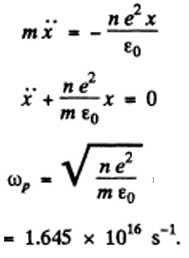2: An oscillating circuit consisting of a capacitor with capacitance C and a coil of inductance L maintains free undamped oscillations with voltage amplitude across the capacitor equal to Vm. For an arbitrary moment of time find the relation between the current I in the circuit and the voltage V across the capacitor. Solve this problem using Ohm's law and then the energy conservation law.

Solution:

1. Since there are no sources of emf in the circuit, Ohm’s 1 law reads

q/c = -L(dI/dt)

Where q = change on the capacitor, I = dq/dt = current through the coil. Then

d2q/dt2 + ωo2q = 0, ωo2 = 1/LC

The solution of this equation is,

q = qm cos(ωot + α)

We know, vm = qm/C then

I = -ωo CVm sin(ωot + α)

V = Vm cos(ωot - α)

V2 + I2/(ωo2C2) = V2m

V2 + L2I2/C = V2m

By energy conservation,(1/2)LI2 + q2/2C = constant

When the P.D. across the capacitor takes its maximum value Vm , the current I must be zero. Thus "constant” = (1/2)Vm2

Hence, ,(1/C)LI2 + V2 = Vm2 .

3: An oscillating circuit consists of a capacitor with capacitance C, a coil of inductance L with negligible resistance, and a switch. With the switch disconnected, the capacitor was charged to a voltage Vm, and then at the moment t =0 the switch was closed. Find:

(a) the current I (t) in the circuit as a function of time;

(b) the emf of self-inductance in the coil at the moments when the electric energy of the capacitor is equal to that of the current in the coil.

Solution:

1. After the switch was closed, the circuit satisfies

-L(dI/dt) = q/C

d2q/dt2 + ωo2q = 0 => q = CVm cos(ωot)

Where we have used the fact that when the switch is closed, we must have

V=q/C = Vm, I = dq/dt = 0 at t=0.

(a)I = aq/dt = -CVm ωo sin ωot = -Vm √(C/L) sin ωot

(b)The electrical energy of the capacitor is q2/2C α cos2ωot and of the inductor is (1/2)LI2 α sin2ωot

The two are equal when ωot = π/4

At that instant the emf of the self-inductance is -L (di/dt) = Vmcosωot = Vm/√2.

4: In an oscillating circuit consisting of a parallel-plate capacitor and an inductance coil with negligible active resistance the oscillations with energy W are sustained. The capacitor plates were slowly drawn apart to increase the oscillation frequency η-fold. What work was done in the process?

Solution:

1. In the oscillating circuit, let q = qm cosωt be the change on the condenser where ω2 = (1/LC) and C is the instantaneous capacity of the condenser (S = area of plates)

C = εoS/y

y = distance between the plates. Since the oscillation frequency increases η fold, the quantity ω2 = y/ εoS L changes η2 fold and so does y i.e. changes from y0 initially to η2y0 finally. Now the P.D. across the condenser is :

V = (qm/C) cosωt = (yqmoS )cosωt

And hence the electric field between the plates is T

E = (qmoS )cosωt

Thus, the change on the plate being qm cosωt , the force on the plate is

F = (q2moS )cos2ωt

Since this force is always positive and the plate is pulled slowly we can use the average force is qm2/2εoS

And work done is A = (η2 – 1) qm2yo/2εoS

But , qm2yo/2εoS = qm2/2Co =W is the initial stored energy.

Therefore, A = (η2 – 1)W.

5: In an oscillating circuit shown in Figure below the coil inductance is equal to L = 2.5 mH and the capacitors have capacitances C1= 2.0 μF and C2 = 3.0 μF. The capacitors were charged to a voltage V = 180 V, and then the switch Sw was closed. Find: (a) the natural oscillation frequency; (b) the peak value of the current flowing through the coil.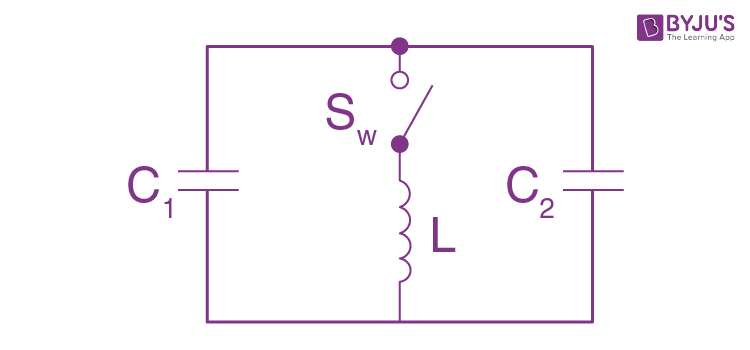Solution:

1.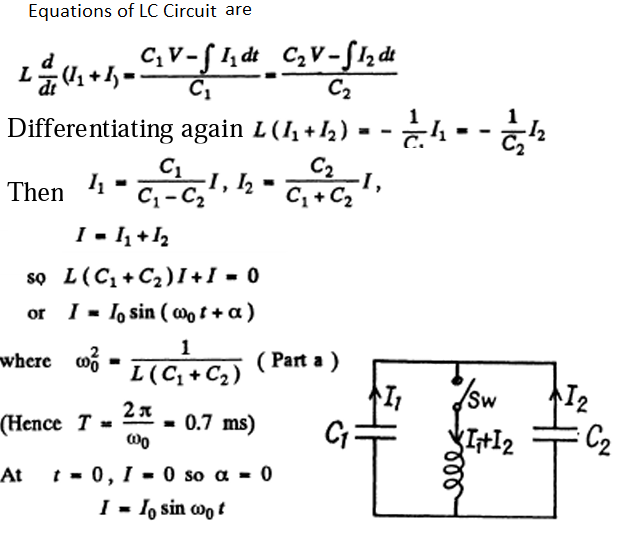The peak value of the current is 70 and it is related to the voltage V by the first equation, LI = V – $\int Idt/(C_1+C_2)$

Or LωoIo cosωot = V – (1/(c1+c2) $\int_0^1 I_0 sinω_0t\ dt$

(The P.D. across the inductance is V at t = 0)

= V + 1/(C1+C2). Ioo(cos ωot – 1)

Hence, I0 = (C1 + C2) ωoV = V√[(C1 + C2)/L] = 8.05 A.

6: An electric circuit shown in Figure below has a negligibly small active resistance. The left-hand capacitor was charged to a voltage V0 and then at the moment t = 0 the switch Sw was closed. Find the time dependence of the voltages in left and right capacitors.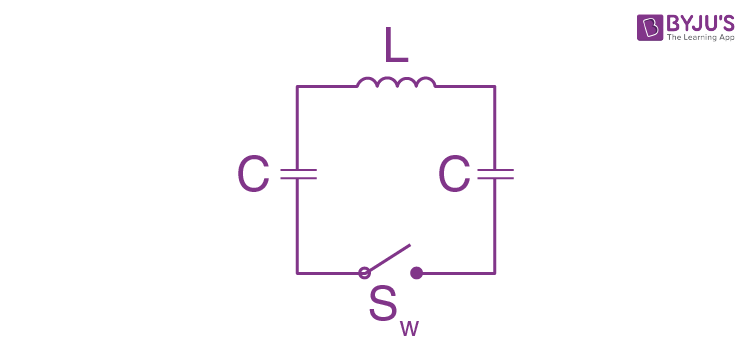Solution:

1. Initially q1 = C V0 and q2 = 0. After the switch is closed change flows and we get,

q1 + q2 = Cv0

q1/C + L(dI/dt) - q2/C = 0

Also, $I =\dot{q_1} = - \dot{q_2}$

$L \ddot{I} + \frac{2I}{C} = 0$

Hence, $\ddot{I} + \omega_0^2 I = 0, \omega_0^2 = \frac{2}{LC}$

The solution of this equation subject to

I = 0 at t = 0 is I = I0 sinωot.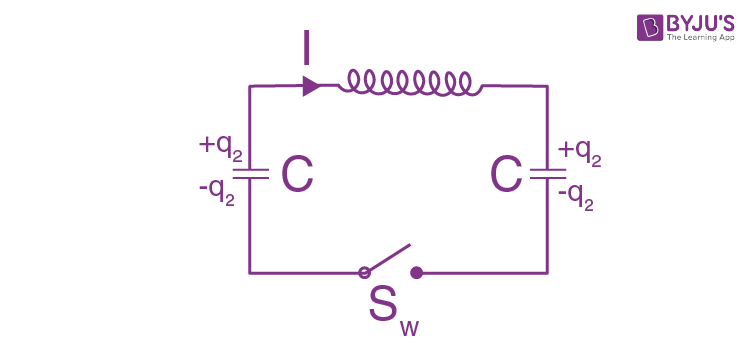On integrating, we have

q1 = A – (I0o) cosωot and q2 = B + (I0o) cosωot

Therefore, (A-B)/C – (2I0oC) cosωot + LI0ωo cosωot = 0

A = B = CV0/2 and CV0/2 + I00 = 0

So, q1 = CV0/2 (1 + cosωot) and q2 = CV0/2 (1 - cosωot)

7: An oscillating circuit consists of an inductance coil L and a capacitor with capacitance C. The resistance of the coil and the lead wires is negligible. The coil is placed in a permanent magnetic field so that the total flux passing through all the turns of the coil is equal to φ. At the moment t = 0 the magnetic field was switched off. Assuming the switching off time to be negligible compared to the natural oscillation period of the circuit, find the circuit current as a function of time t.

Solution:

1. The flux in the coil is

ϕ(t) = ϕ for t < 0 and ϕ(t) = 0 for t > 0

The equation of the current is $-L(dI/dt) = \frac{\int_0^1Idt}{C}$

...(1)

This shows, LC (d2I/dt2) + I = 0 or with ω02 = 1/LC

I = Io sin(ωot + α)

Putting values in (1) -LIo ω0 cos(ωot + α) = -Io0C[cos(ωot + α) – cos α

This implies, cos α = 0, therefore, I = Io cos(ωot) and , I = - Io cos(ωot)

From Faraday’s law: ε = -dϕ/dt = -L(dI/dt)

Or integrating form, t = - ε to – ε where ε ->0

ϕ = LI0 with + sign in I or I = (ϕ/L) cos(ωot)

8: The free damped oscillations are maintained in a circuit, such that the voltage across the capacitor varies as V = Vmθ-βt cos ωt.

Find the moments of time when the modulus of the voltage across the capacitor reaches (a) peak values; (b) maximum (extremum) values.

Solution:

1. (a) Given The phrase ‘peak values’ is not clear. The answer is obtained on taking |cos ωt| = 1 i.e. t = πn/ω.

(b) For extrema, dV/dt = 0

-β cosωt – ω sinωt = 0

tan ωt = -β/ω

ωt = nπ + tan-1(-β/ω).

9: A certain oscillating circuit consists of a capacitor with capacitance C, a coil with inductance L and active resistance R, and a switch. When the switch was disconnected, the capacitor was charged; then the switch was closed and oscillations set in. Find the ratio of the voltage across the capacitor to its peak value at the moment immediately after closing the switch.

Solution:

1. The equation of the circuit is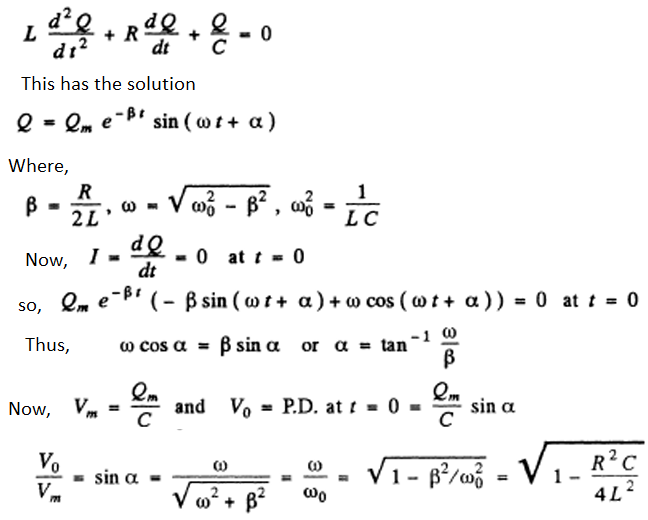10: A circuit with capacitance C and inductance L generates free damped oscillations with current varying with time as I = lm e-βt sin ωt. Find the voltage across the capacitor as a function of time, and in particular, at the moment t = 0.

Solution:

1. -dQ/dt = I = Im e-βt sin ωt

= gm Im e-βt+iωt (gm means imaginary part)

Then,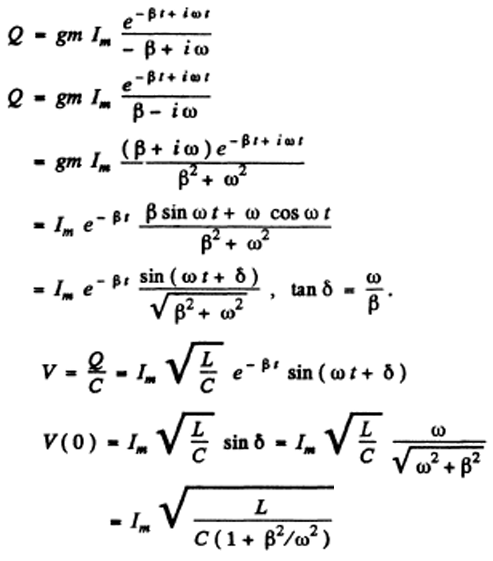11: An oscillating circuit consists of a capacitor with capacitance C = 4.0 μF and a coil with inductance L = 2.0 mH and active resistance R = 10Ω. Find the ratio of the energy of the coil's magnetic field to that of the capacitor's electric field at the moment when the current has the maximum value.

Solution:

1. I = Im e-βt sin ωt

Β = R/2L, ω0 = √(1/LC) and ω = √(ω02 – β2)

I = $-\dot{q}$, q = charge on the capacitor

Then, q = Im e-βt [sin (ωt+ δ)/√(ω2 + β2)] and tan δ = ω/β

Thus, WM = (1/2)L Im2 e-2βt sin2 ωt

$W_E = \frac{I_m^2}{2C} \frac{e^{-2 \beta t}sin^2(\omega t + \delta)}{\omega^2 + \beta^2} = \frac{LI_m^2}{2}e^{-2\beta t}sin^2(\omega t+ \delta)$

Current is maximum when, d/dt(e-βt) sinωt = 0

-β sin ωt + ω cos ωt = 0

or tan ωt= ω/β = tan δ

that is, ωt = n π + δ

Hence, $\frac{W_M}{W_E} = \frac{sin^2(\omega t)}{ sin^2(\omega t+\delta)}=\frac{1}{4 cos^2\delta}$

= 1/(4 β202) = ω02/4 β2 = 1/LC x L2/R2 = 5

(WM is the magnetic energy of the inductance coil and WE is the electric energy to the capacitor.)

12: An oscillating circuit consists of two coils connected in series whose inductances are L1 and L2, active resistances are R1 and R2, and mutual inductance is negligible. These coils are to be replaced by one, keeping the frequency and the quality factor of the circuit constant. Find the inductance and the active resistance of such a coil.

Solution:

1. L = L1 + L2, R = R1 + R2.

13: How soon does the current amplitude in an oscillating circuit with quality factor Q = 5000 decrease η = 2.0 times if the oscillation frequency is v = 2.2 MHz?

Solution:

1. Q = π/βT or β = π/QT

Now, βt = ln η

so, t = (ln η/π) QT = Q ln η/πv = 0.5 ms

14: An oscillating circuit consists of capacitance C = 10μF, inductance L = 25 mH, and active resistance R = 1.0 Ω. How many oscillation periods does it take the current amplitude to decrease e-fold?

Solution:

1. Current decreases e fold in time

t = 1/βt = 2L/R sec = 2L/RT oscillations

= 2L/R (ω/2 π)

= $\frac{L}{\pi R}\sqrt{\frac{L}{C}-\frac{R^2}{4L^2}} = \frac{1}{2 \pi}\sqrt{\frac{4L}{R^2C}-1}$

= 15.9 oscillations

15: How much (in percent) does the free oscillation frequency ω of a circuit with quality factor Q = 5.0 differ from the natural oscillation frequency ω0 of that circuit?

Solution:

1.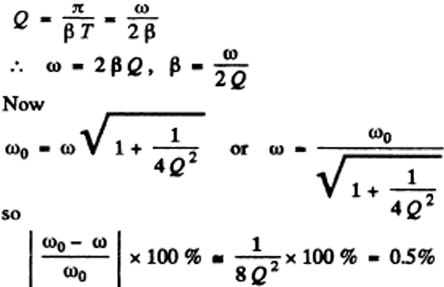16: In a circuit shown in figure below the battery emf is equal to 2.0 V, its internal resistance is r = 9.0Ω, the capacitance of the capacitor is C = 10μF, the coil inductance is L = 100 mH, and the resistance is R = 1.0 Ω. At a certain moment the switch Sw was disconnected. Find the energy of oscillations in the circuit

(a) immediately after the switch was disconnected;

(b) t = 0.30 s after the switch was disconnected.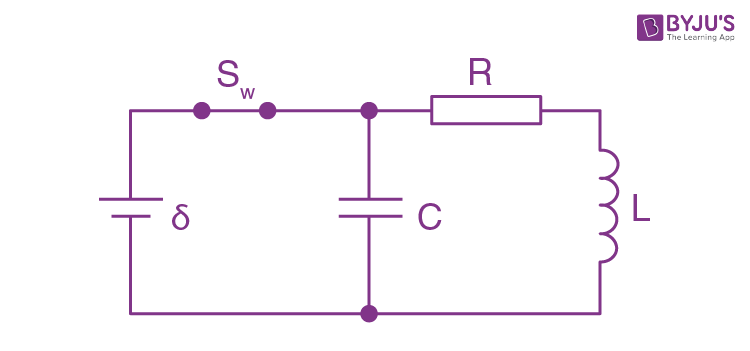Solution:

1. At t = 0 current through the coil = ε/(R+r)

P.D. across the condenser = ε/(R+r)

(a) At t = 0, energy stored = W0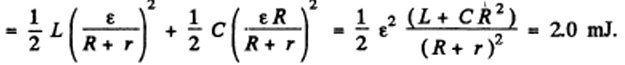(b) The current and the change stored decrease as e-tR/2L so energy decreases as e-tR/L

W = W0 e-tR/L = 0.10 mJ

17: Damped oscillations are induced in a circuit whose quality factor is Q = 50 and natural oscillation frequency is v0 = 5.5 kHz. How soon will the energy stored in the circuit decrease η = 2.0 times?

Solution:

1.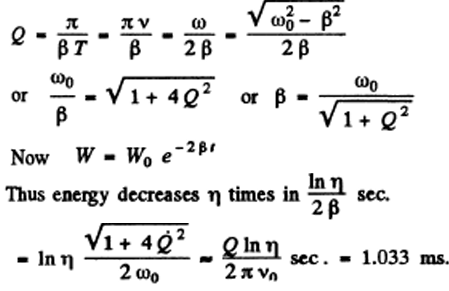18: An oscillating circuit incorporates a leaking capacitor. Its capacitance is equal to C and active resistance to R. The coil inductance is L. The resistance of the coil and the wires is negligible. Find:

(a) The damped oscillation frequency of such a circuit;

(b) its quality factor.

Solution:

1. In a leaky condenser

dq/dt = I-I’ where I’ = V/R = leak current

Now, V = q/c = -L (dI/dt) = -L (d/dt)(dq/dt + V/R)

= -L(d2q/dt2) – L/RC (dq/dt)

Or $\ddot{q} + \frac{1}{RC}\frac{dQ}{dt}+\frac{1}{LC}q = 0$

Then, q = qme-βtsin(ωt +α)

(a) β = 1/2RC, ω02 = 1/LC and ω = √(ω02 – β2)

= √(1/LC – 1/4R2C2)

(b) Q = ω/2 β = RC √(1/LC – 1/4R2C2)

= (1/2) √(4R2C/L - 1)

19: Find the quality factor of a circuit with capacitance C = 2.0 μF and inductance L = 5.0 mH if the maintenance of undamped oscillations in the circuit with the voltage amplitude across the capacitor being equal to Vm = 1.0 V requires a power (P) 0.10 mW. The damping of oscillations is sufficiently low.

Solution:

1. Given, V = Vme-βtsin(ωt), ω = ω0βT << 1

Power loss = (energy loss per cycle)/T

= (1/2) CVm2 x 2β

(energy decreases as W0 e-2βt so loss per cycle is W0 x 2βT)

Thus, <p> = (1/2) CVm2 x R/L

Or R = 2<p>/ Vm2 x L/C

Hence, Q = (1/R) √(L/C) = √(C/L) Vm2/2<p> = 100 (on substituting values)

20: What mean power should be fed to an oscillating circuit with active resistance R = 0.45Ω to maintain undamped harmonic oscillations with current amplitude Im = 30 mA?

Solution:

1. Energy is lost across the resistance and the mean power lass is

= R<I2> = (1/2)RIm2 = 0.2 mW.

This power should be fed to the circuit to maintain undamped oscillations.